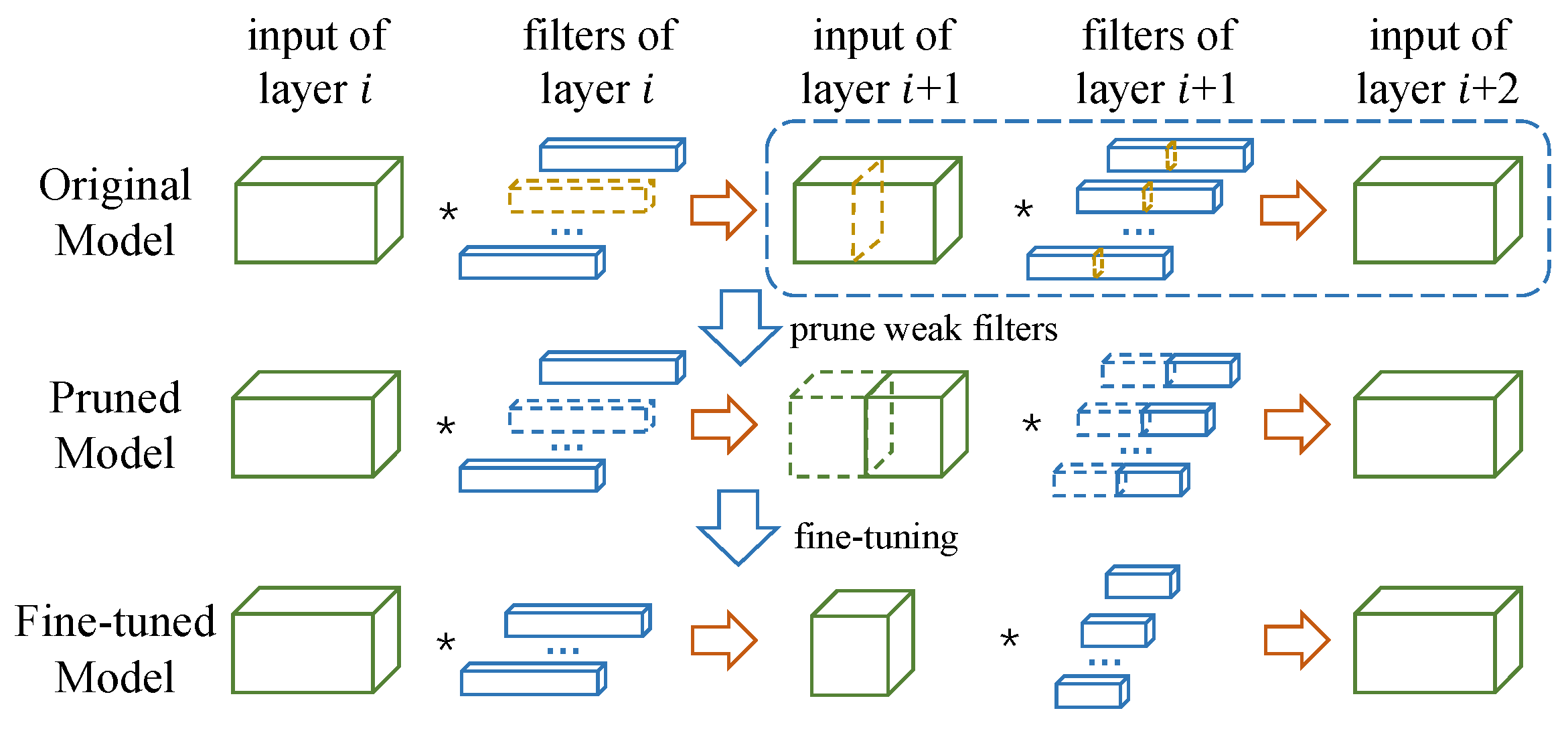# 1. 概览

## 1.1 ThiNet框架## 1.2 收集训练样本\begin{equation}
y = \sum_{c=1}^C\sum_{k_1=1}^K\sum_{k_2=1}^K\widehat{\mathcal{W}}_{c,k_1,k_2}\times x_{c,k_1,k_2}+b
\end{equation}

\begin{equation*}
\hat{x}_c=\sum_{k_1=1}^K\sum_{k_2=1}^K\widehat{\mathcal{W}}_{c,k_1,k_2}\times x_{c,k_1,k_2}
\end{equation*}

\begin{equation}
\hat{y} = \sum_{c \in S}\hat{x}_c
\end{equation}

## 1.3 一种用于通道选择的贪心算法

\begin{equation}
\begin{aligned}
\mathop{\arg\min}\limits_{S}  & \sum_{i=1}^{m}\left(\hat{y}_i-\sum_{j\in S}\mathbf{\hat{x}}_{i,j}\right)^2\\
\end{aligned}
\end{equation}

\begin{equation}
\begin{aligned}
\mathop{\arg\min}\limits_{T} & \sum_{i=1}^{m}\left(\sum_{j\in T}\mathbf{\hat{x}}_{i,j}\right)^2\\
\end{aligned}
\end{equation}

## 1.4 最小化重构误差

\begin{equation}
\mathbf{\hat{w}}=\mathop{\arg\min}\limits_{\mathbf{w}}\sum_{i=1}^{m}(\hat{y}_i- \mathbf{w}^\mathrm{T}\mathbf{\hat{x}}^*_i)^2.
\end{equation}

# 2. 实验结果

## 2.1 不同的滤波器选择策略## 2.2 VGG16在ImageNet上的表现

Model Top-1 Top-5 #Param. #FLOPs f./b. (ms)
Original1 68.34% 88.44% 138.34M 30.94B 189.92/407.56
ThiNet-Conv 69.80% 89.53% 131.44M 9.58B 76.71/152.05
Train from scratch 67.00% 87.45% 131.44M 9.58B 76.71/152.05
ThiNet-GAP 67.34% 87.92% 8.32M 9.34B 71.73/145.51
ThiNet-Tiny2 59.34% 81.97% 1.32M 2.01B 29.51/55.83
SqueezeNet  57.67% 80.39% 1.24M 1.72B 37.30/68.62

1为公平比较，原VGG-16模型是使用单张中心裁剪（先缩放至256$\times$256）的图片测试所得，该测试方法被广泛使用于[10, 14]。因此，由这种方法测试所得的准确度低于某些报告中的结果

2组织ImageNet数据集时，通常有两种方法来对图像进行缩放：

1. 固定大小： 每张图首先缩放至256$\times$256，再中心裁剪得到224$\times$224大小的区域；
2. 保持长宽比： 每张图首先将短边缩放至256，再中心裁剪；

Model Top-1 Top-5 #Param. $\downarrow$ #FLOPs $\downarrow$
APoZ-1  -2.16% -0.84% 2.04$\times$ $\approx$1$\times$
APoZ-2  +1.81% +1.25% 2.70$\times$ $\approx$1$\times$
Taylor-1  - -1.44% $\approx$1$\times$ 2.68$\times$
Taylor-2  - -3.94% $\approx$1$\times$ 3.86$\times$
ThiNet-WS  +1.01% +0.69% 1.05$\times$ 3.23$\times$
ThiNet-Conv +1.46% +1.09% 1.05$\times$ 3.23$\times$
ThiNet-GAP -1.00% -0.52% 16.63$\times$ 3.31$\times$

# 3. 其他结果

## 3.1 剪枝过程的计算代价

1. 前者的计算速度取决于模型的预测速度，在M40上使用VGG-16花费了769.37s。
2. 后者的计算速度由当前层的滤波器数量决定（conv1_1层是64，conv4_3层是512)。我们测试了这两层的计算时间，分别需要2.34s137.36s来特征选择。

## 3.2 微调的作用

Method Before fine-tuning After fine-tuning
random 51.26% 67.83%
weight sum  40.99% 63.72%
APoZ  58.16% 68.78%
ThiNet w/o w 68.24% 70.38%
ThiNet 70.75% 71.11%

# 参考文献

• Jian-Hao Luo, Jianxin Wu, and Weiyao Lin: ThiNet: A Filter Level Pruning Method for Deep Neural Network Compression

• To appear in International Conference on Computer Vision (ICCV), 2017.

• @inproceedings{iccv2017ThiNet,
title={{ThiNet: A Filter Level Pruning Method for Deep Neural Network Compression}},
author={Jian-Hao Luo, Jianxin Wu, and Weiyao Lin},
booktitle={International Conference on Computer Vision (ICCV)},
month={October},
year={2017}
}